# Cramers Rule Gabriel Cramer was a Swiss mathematician

• Slides: 21Cramer's Rule Gabriel Cramer was a Swiss mathematician (17041752) Reference from: http: //www. mathcentre. ac. uk : Fundamentals Methods of Mathematical Economics 4 th Edition (Page 103 -107)Introduction o Cramer’s Rule is a method for solving linear simultaneous equations. It makes use of determinants and so a knowledge of these is necessary before proceeding. o Cramer’s Rule relies on determinants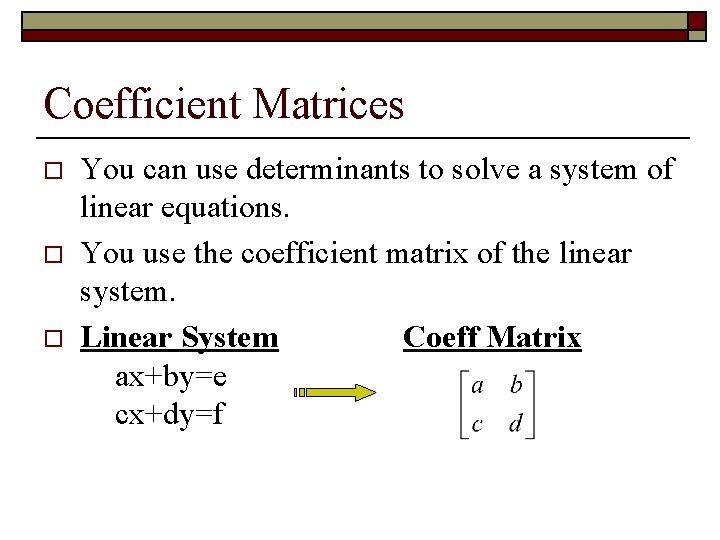Coefficient Matrices o o o You can use determinants to solve a system of linear equations. You use the coefficient matrix of the linear system. Linear System Coeff Matrix ax+by=e cx+dy=f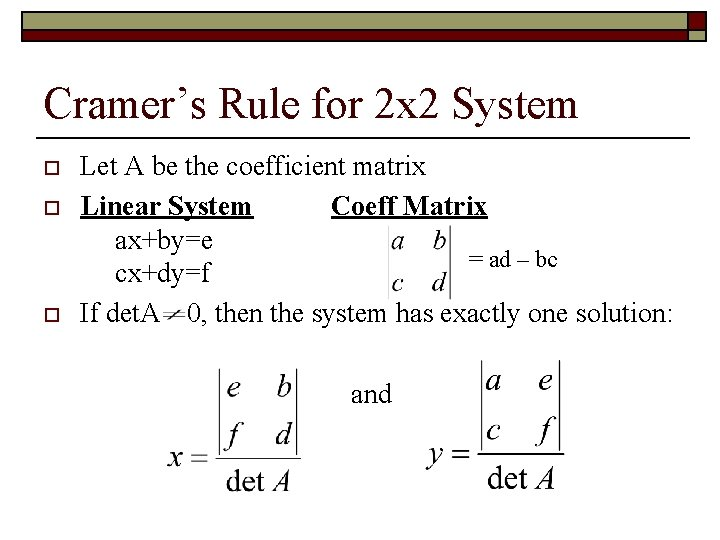Cramer’s Rule for 2 x 2 System o o o Let A be the coefficient matrix Linear System Coeff Matrix ax+by=e = ad – bc cx+dy=f If det. A 0, then the system has exactly one solution: and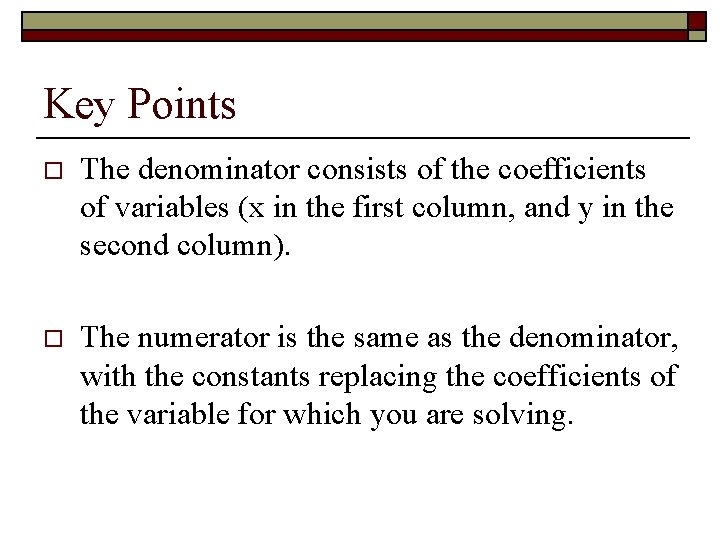Key Points o The denominator consists of the coefficients of variables (x in the first column, and y in the second column). o The numerator is the same as the denominator, with the constants replacing the coefficients of the variable for which you are solving.Example - Applying Cramer’s Rule on a System of Two Equations Solve the system: o 8 x+5 y= 2 o 2 x-4 y= -10 The coefficient matrix is: and So: andSolution: (-1, 2)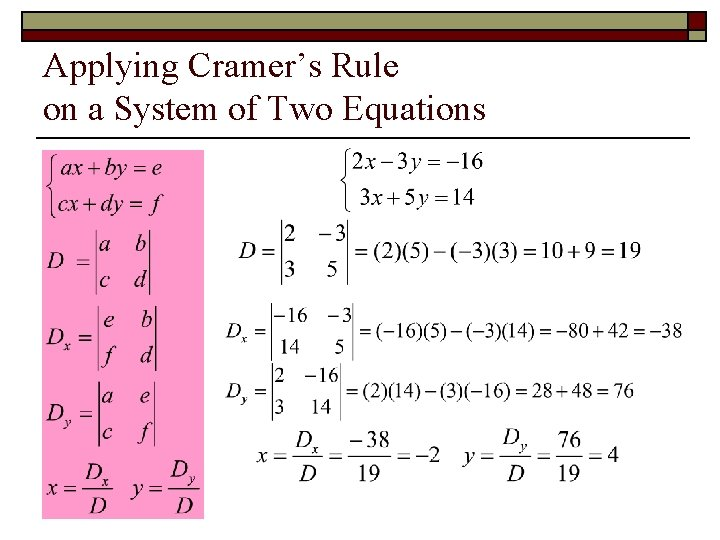Applying Cramer’s Rule on a System of Two Equations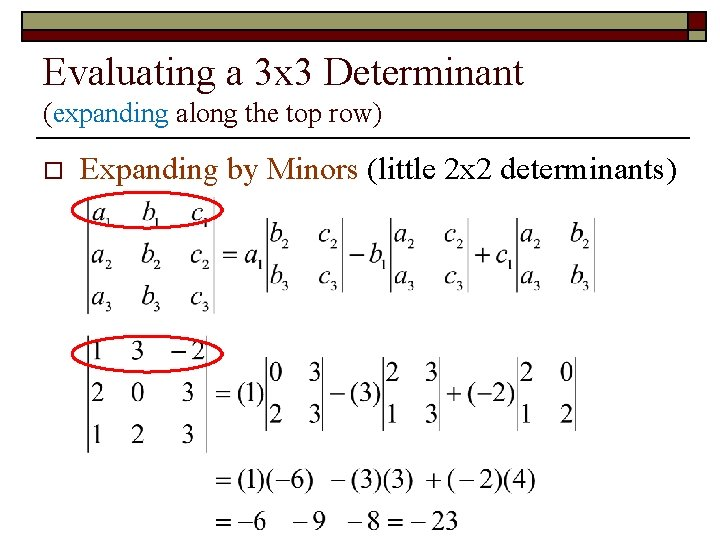Evaluating a 3 x 3 Determinant (expanding along the top row) o Expanding by Minors (little 2 x 2 determinants)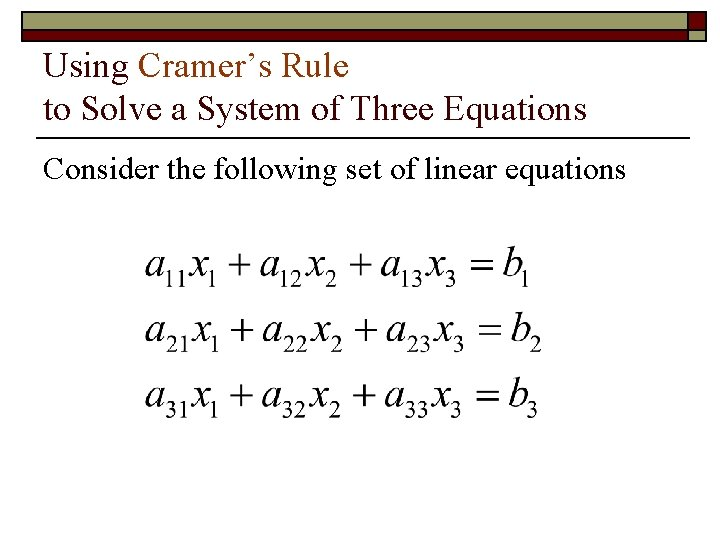Using Cramer’s Rule to Solve a System of Three Equations Consider the following set of linear equations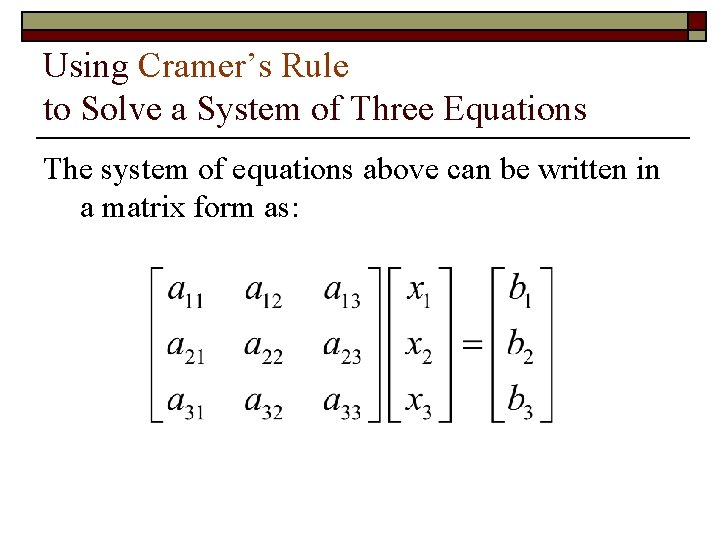Using Cramer’s Rule to Solve a System of Three Equations The system of equations above can be written in a matrix form as: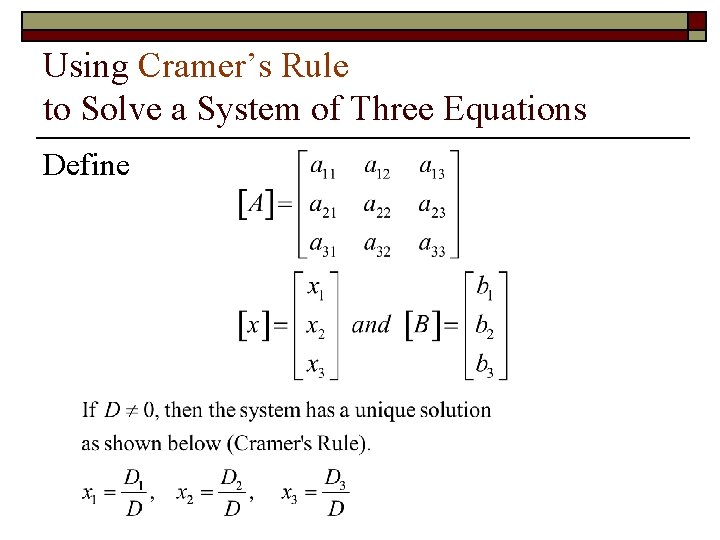Using Cramer’s Rule to Solve a System of Three Equations Define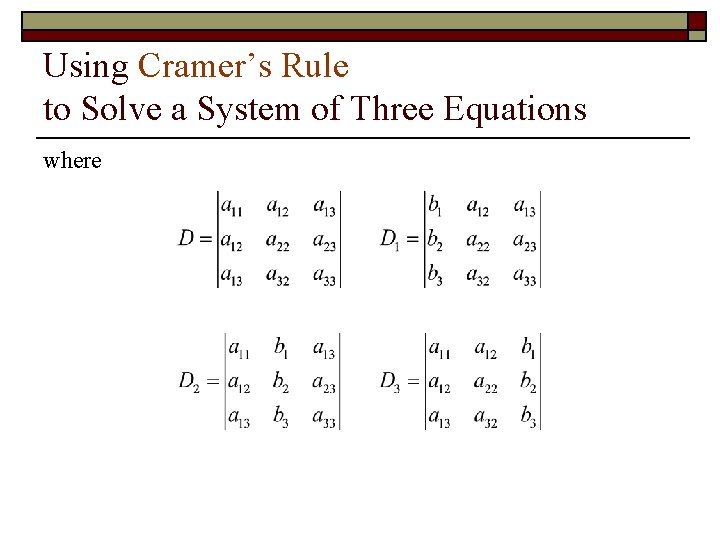Using Cramer’s Rule to Solve a System of Three Equations whereExample 1 Consider the following equations: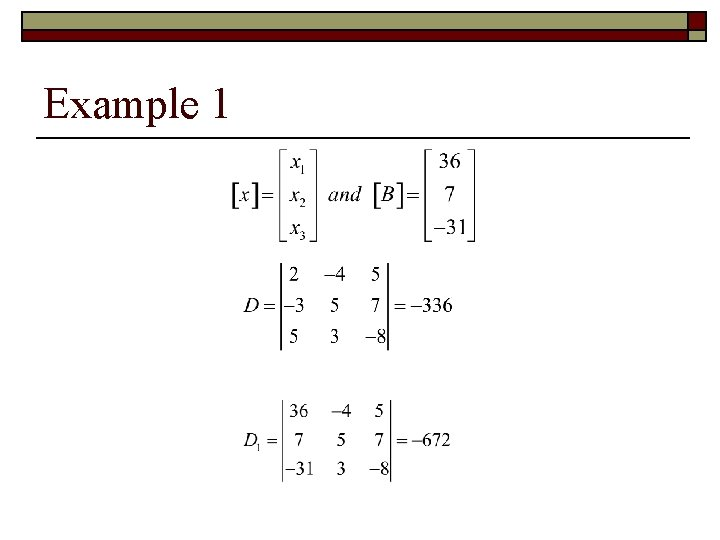Example 1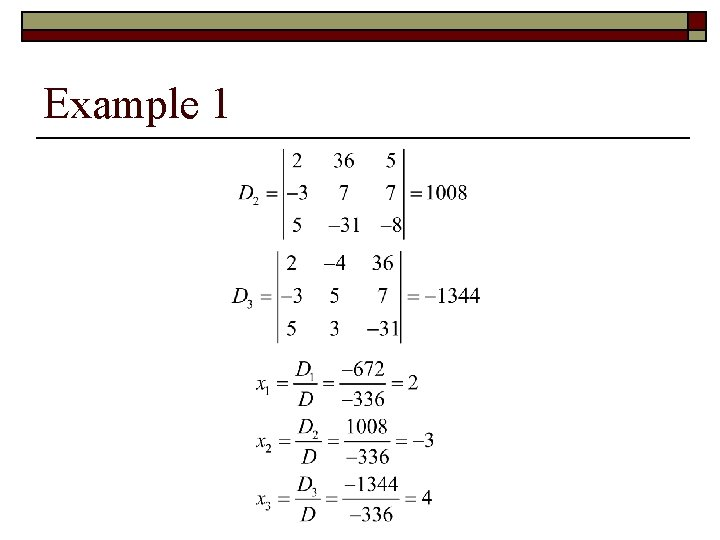Example 1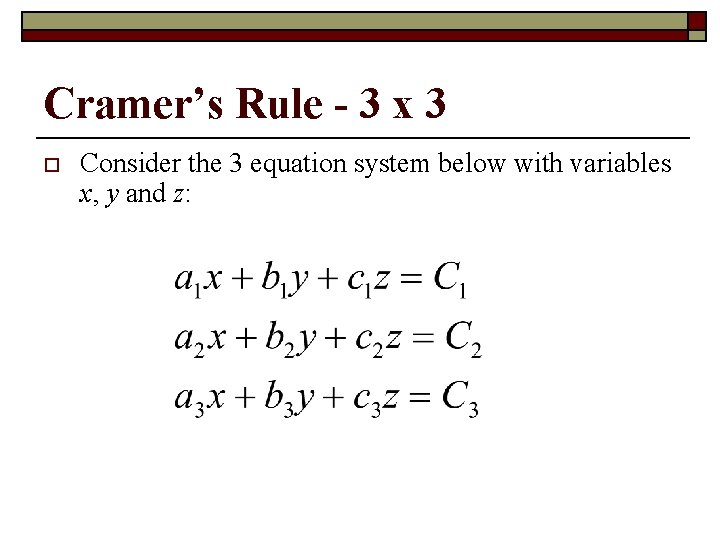Cramer’s Rule - 3 x 3 o Consider the 3 equation system below with variables x, y and z: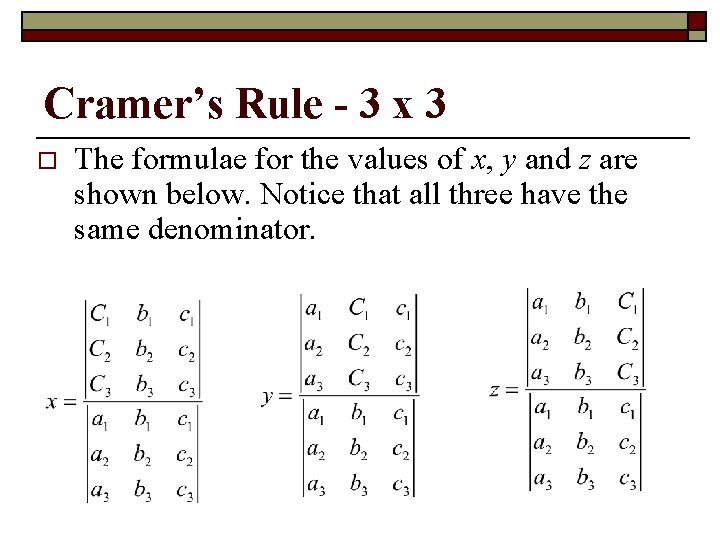Cramer’s Rule - 3 x 3 o The formulae for the values of x, y and z are shown below. Notice that all three have the same denominator.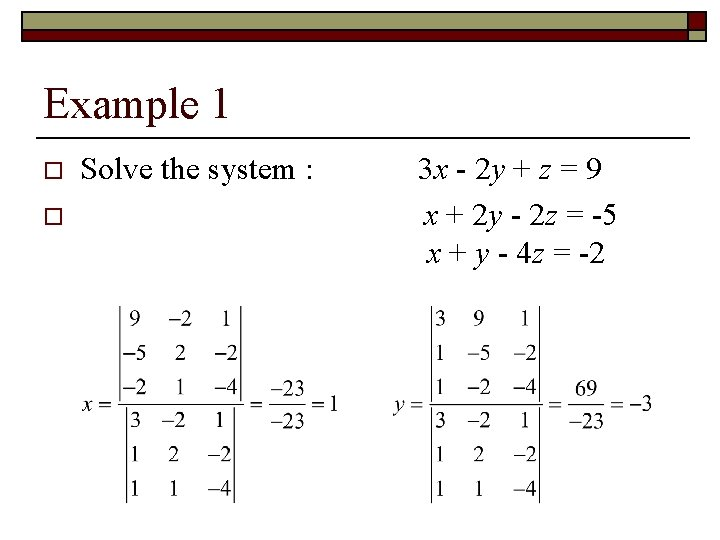Example 1 o o Solve the system : 3 x - 2 y + z = 9 x + 2 y - 2 z = -5 x + y - 4 z = -2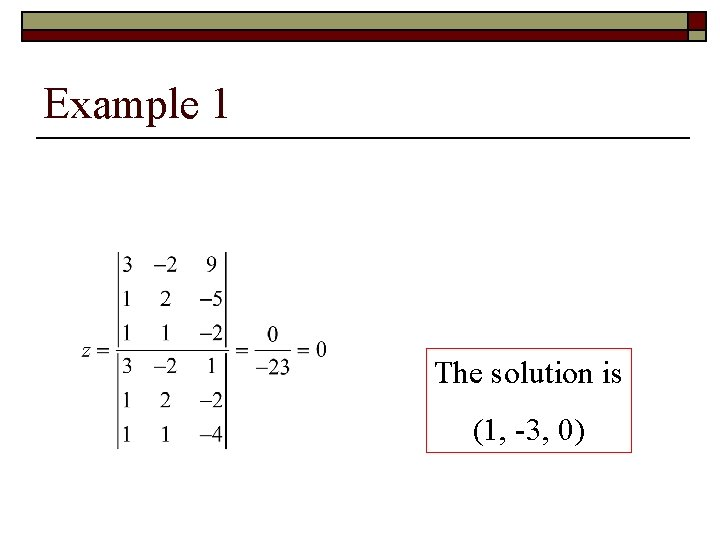Example 1 The solution is (1, -3, 0)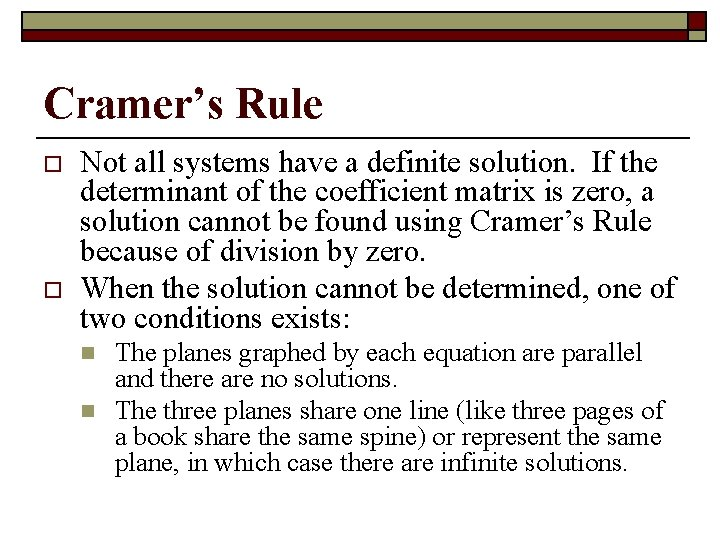Cramer’s Rule o o Not all systems have a definite solution. If the determinant of the coefficient matrix is zero, a solution cannot be found using Cramer’s Rule because of division by zero. When the solution cannot be determined, one of two conditions exists: n n The planes graphed by each equation are parallel and there are no solutions. The three planes share one line (like three pages of a book share the same spine) or represent the same plane, in which case there are infinite solutions.